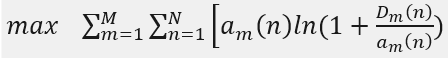# Cvx DCP error:%{real affine} .* {convex}

a(m,n) is variable,which size is(M,N).D(m,n) is constant.In my code,I express it as follows:

``````  R1(m,n)=a(m,n)*inv_pos(D(m,n))*(rel_entr(D(m,n)+a(m,n),a(m,n))+rel_entr(a(m,n),D(m,n)+a(m,n)));
``````

But there are some errors: Illegal expression:{real affine} .* {convex}
How shoud I solve it? thank you very much!

`a*log(1+D/a)` can be rewritten as `-rel_entr(a,a+D)`

I’ll let you deal with the indexing.

If you have access to Mosek, use that as solver, with CVX 2.2. Otherwise, install CVXQUAD’s exponential.m replacement CVXQUAD: How to use CVXQUAD's Pade Approximant instead of CVX's unreliable Successive Approximation for GP mode, log, exp, entr, rel_entr, kl_div, log_det, det_rootn, exponential cone. CVXQUAD's Quantum (Matrix) Entropy & Matrix Log related functions .